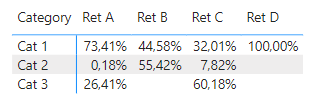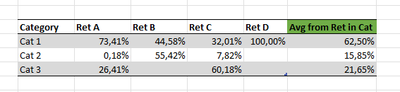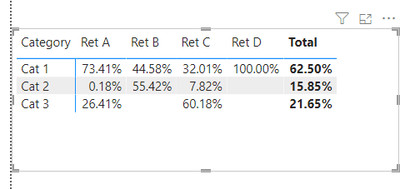cancel
Showing results for
Did you mean:Helper I

## Need help with the calculation of averages

Hi all,
I have a table with retailers, categories and values.To calculate the percentage of categories per retailer, I am using a measure from this forum.Ret % =

Var _N1=[TT Sum]

Var _N2=CALCULATE(SUM(Data[Value]),FILTER(ALL(Data),[Retailer]=SELECTEDVALUE(DimRetailer[Retailer])))

Return

DIVIDE(_N1,_N2)

Now I am still at a loss how to calculate the average of all retailers per category from the result of the measure.Any help is appreciated!

1 ACCEPTED SOLUTIONHelper I

This is exactly what I was looking for, thank you so much!
I have adjusted the formula with AVERAGEX and the results are perfect.

`Measure = VAR _1 =    SUMMARIZE ( DimRetailer, DimRetailer[Retailer], "aaa", [Ret %] )RETURN    IF ( ISINSCOPE ( DimRetailer[Retailer] ), [Ret %], AVERAGEX( _1, [aaa] ) )`
2 REPLIES 2Community Support

Hi @MBreden ,

Create another measure.

``````Measure =
VAR _1 =
SUMMARIZE ( DimRetailer, DimRetailer[Retailer], "aaa", [Ret %] )
RETURN
IF ( ISINSCOPE ( DimRetailer[Retailer] ), [Ret %], SUMX ( _1, [aaa] ) / 4 )
``````If it does not help, please provide more details with your desired output and pbix file without privacy information (or some sample data) .

Best Regards
Community Support Team _ Rongtie

If this post helps, then please consider Accept it as the solution to help the other members find it more quickly.Helper I

This is exactly what I was looking for, thank you so much!
I have adjusted the formula with AVERAGEX and the results are perfect.

`Measure = VAR _1 =    SUMMARIZE ( DimRetailer, DimRetailer[Retailer], "aaa", [Ret %] )RETURN    IF ( ISINSCOPE ( DimRetailer[Retailer] ), [Ret %], AVERAGEX( _1, [aaa] ) )`